Scene CLIMS: Cross Language Image Matching for

Weakly Supervised Semantic Segmentation

IEEE Conference on Computer Vision and Pattern Recognition (CVPR 2022)

Jinheng Xie1,2,3,4    Xianxu Hou1,2,3,4    Kai Ye1,2,3,4    Linlin Shen1,2,3,4*

1Shenzhen University    2Shenzhen Institute of Artificial Intelligence and Robotics for Society

3Guangdong Key Laboratory of Intelligent Information Processing    4National Engineering Laboratory for Big Data System Computing Technology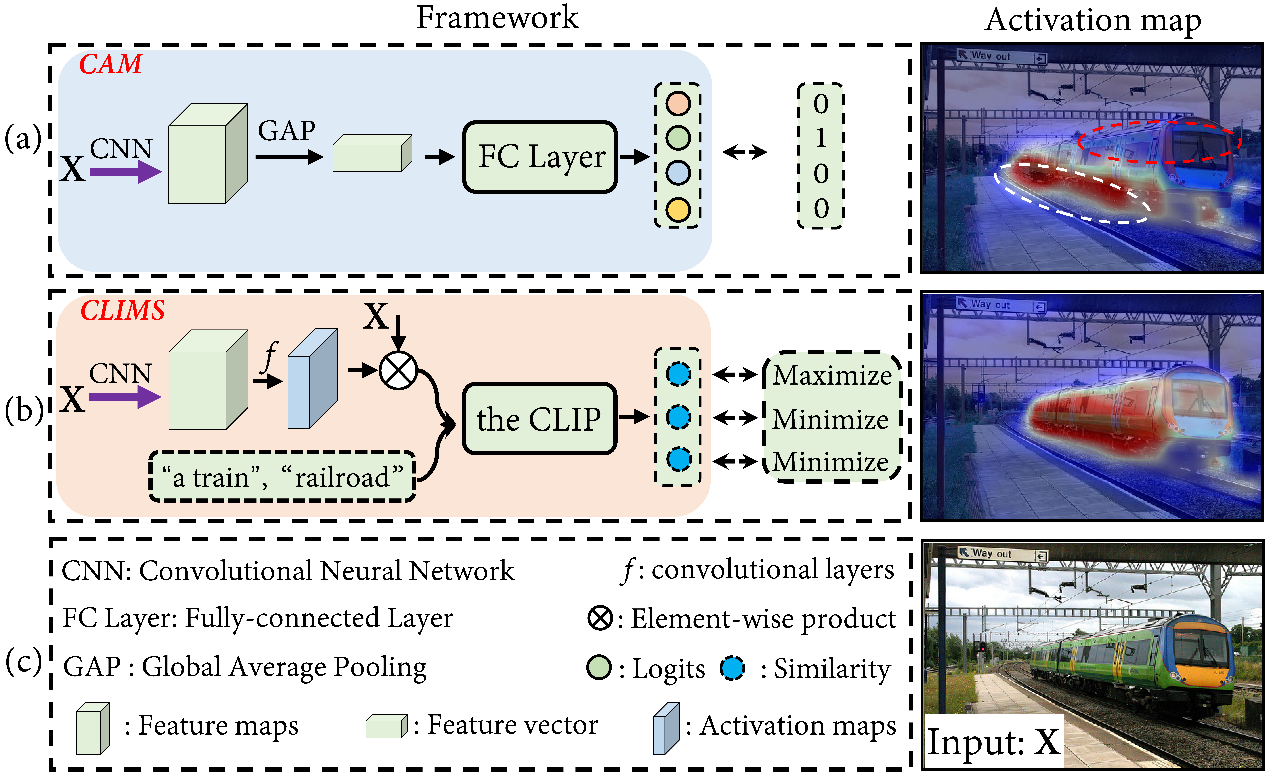Figure 1: (a) Conventional CAM solution. (b) The proposed CLIMS. The problem of false-activation of irrelevant background, e.g., railroad and ground, and underestimation of object contents usually exist in conventional CAM method. To solve this problem, we propose a novel text-driven learning framework, CLIMS, which introduces natural language supervision, i.e., an open-world setting, for exploring complete object contents and excluding irrelevant background regions.

Abstract

It has been widely known that CAM (Class Activation Map) usually only activates discriminative object regions and falsely includes lots of object-related backgrounds. As only a fixed set of image-level object labels are available to the WSSS (weakly supervised semantic segmentation) model, it could be very difficult to suppress those diverse background regions consisting of open set objects. In this paper, we propose a novel Cross Language Image Matching (CLIMS) framework, based on the recently introduced Contrastive Language-Image Pre-training (CLIP) model, for WSSS. The core idea of our framework is to introduce natural language supervision to activate more complete object regions and suppress closely-related open background regions. In particular, we design object, background region and text label matching losses to guide the model to excite more reasonable object regions for CAM of each category. In addition, we design a co-occurring background suppression loss to prevent the model from activating closely-related background regions, with a predefined set of class-related background text descriptions. These designs enable the proposed CLIMS to generate a more complete and compact activation map for the target objects. Extensive experiments on PASCAL VOC2012 dataset show that our CLIMS significantly outperforms the previous state-of-the-art methods.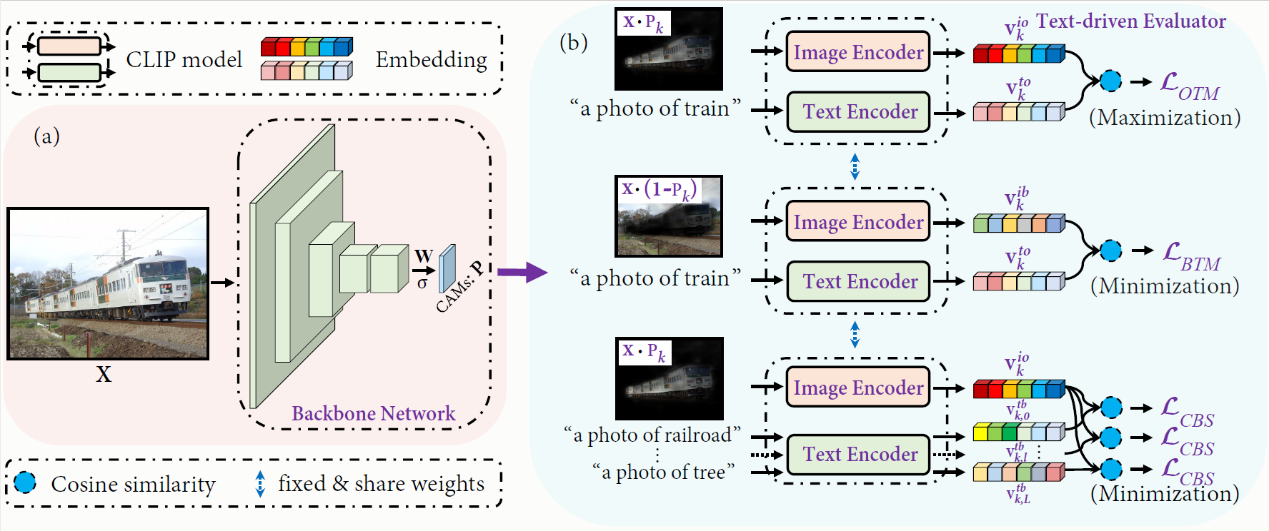Figure 2: An overview of the proposed Cross Language Image Matching framework for WSSS, i.e., CLIMS. (a) The backbone network for predicting initial CAMs. $\sigma$ denotes the sigmoid activation function. $\mW$ denotes the weight matrix of convolutional layers. (b) The text-driven evaluator. It consists of three CLIP-based loss functions, i.e., object region and text label matching loss $\mathcal{L}_{OTM}$, background region and text label matching loss $\mathcal{L}_{BTM}$, and co-occurring background suppression loss $\mathcal{L}_{CBS}$.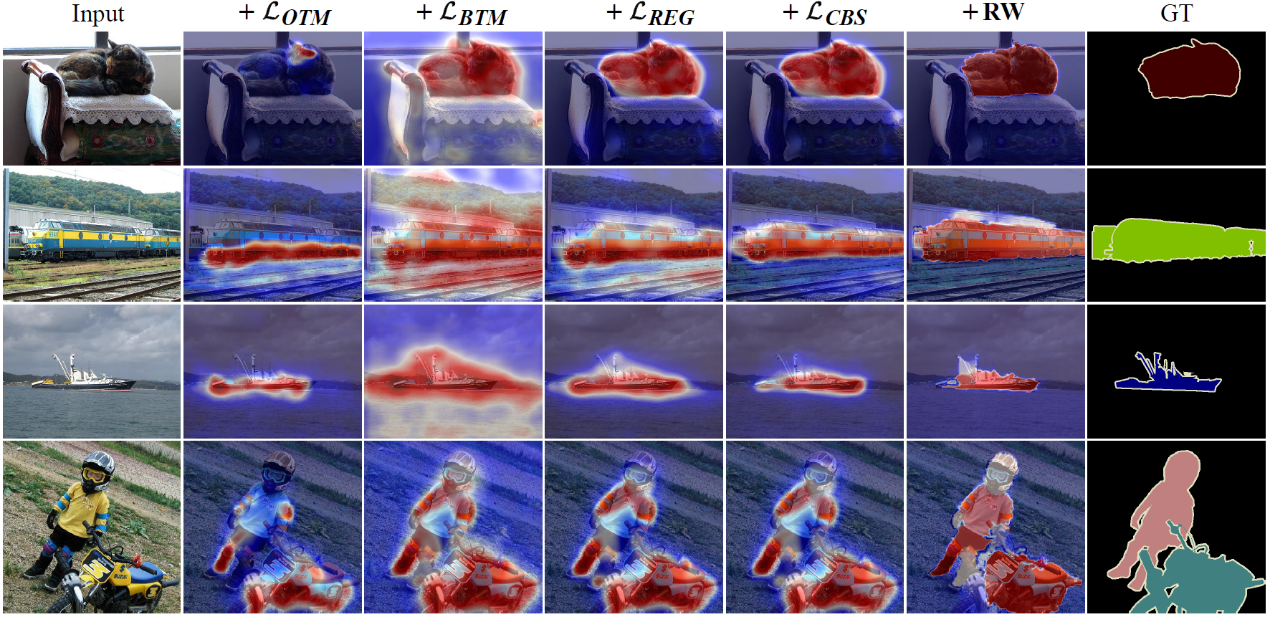Figure 3: Initial CAMs generated by the proposed CLIMS using different combinations of loss functions. Input images are shown in column 1. Columns 2 to 5 present the generated CAMs using $\mathcal{L}_{OTM}$, $\mathcal{L}_{OTM}+\mathcal{L}_{BTM}$, $\mathcal{L}_{OTM}+ \mathcal{L}_{BTM}+\mathcal{L}_{REG}$, and  $\mathcal{L}_{OTM}+\mathcal{L}_{BTM}+\mathcal{L}_{REG}+\mathcal{L}_{CBS}$,  respectively. \textbf{RW} denotes the refinement of PSA~\cite{affinitynet}.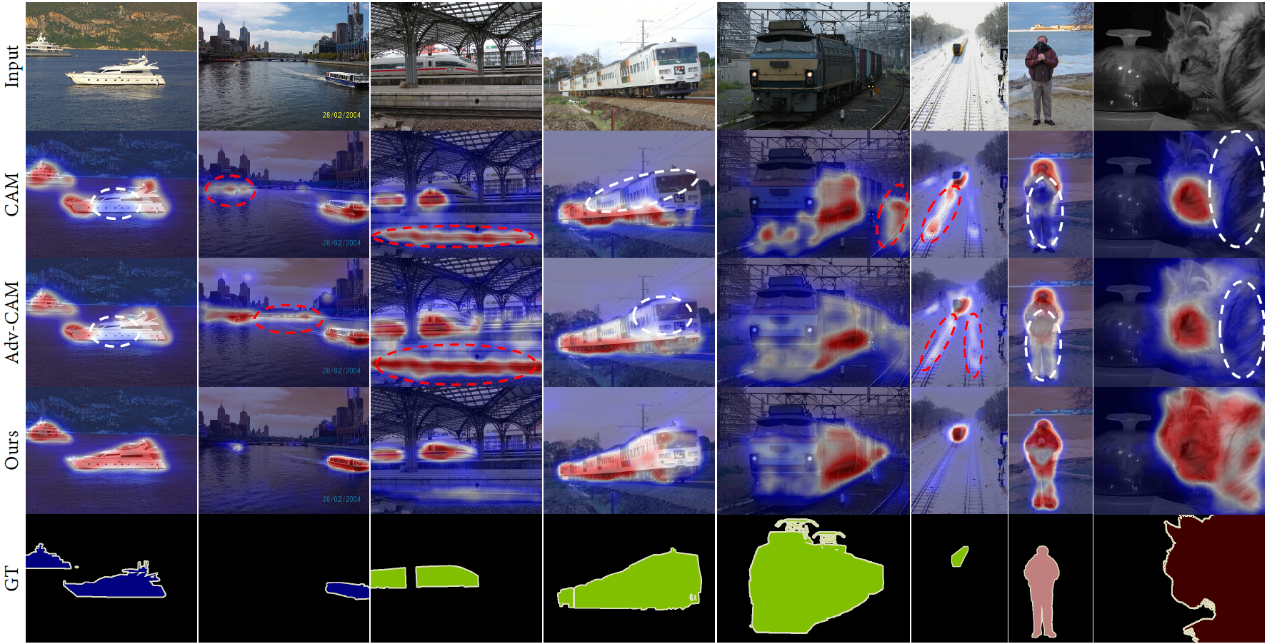Figure 4: Visualization of the initial CAMs generated by CAM, Adv-CAM, and the proposed CLIMS. White dotted circles illustrate the missed object regions. Red dotted circles illustrate the false activation of class-related background regions, e.g., the river and railroad.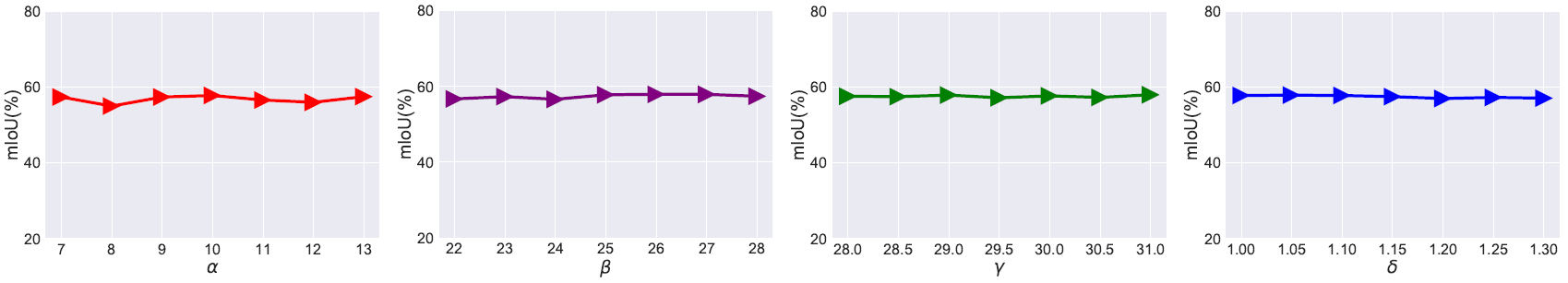Figure 5: Sensitivity analyses of hyper-parameters $\alpha$, $\beta$, $\gamma$, and $\delta$. The mIoU values here are reported on PASCAL VOC2012 \textit{train} set.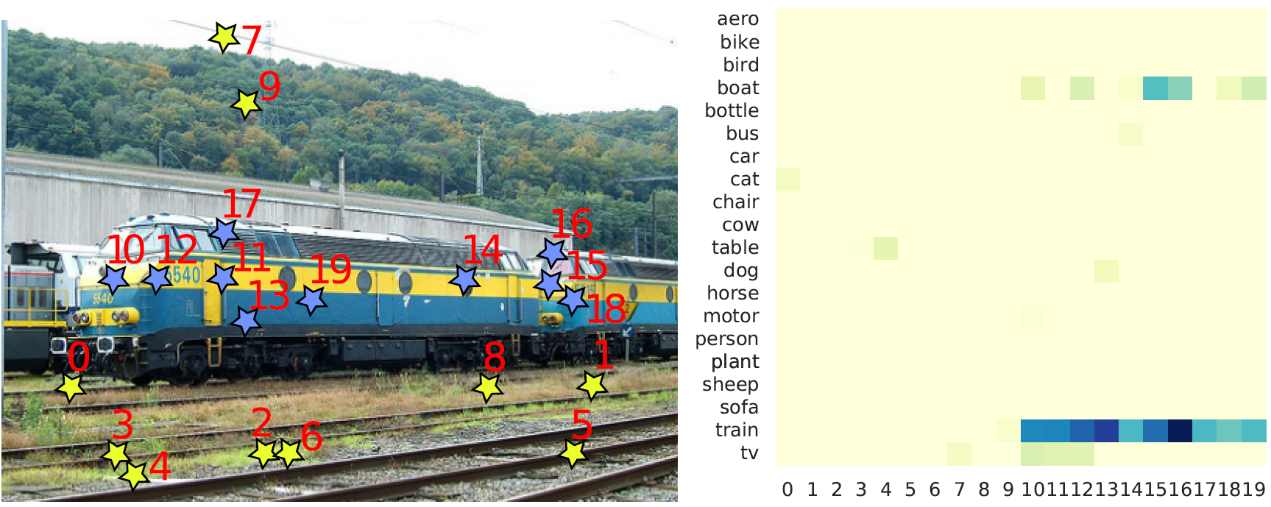Figure 6: Left: the sample image. Yellow and blue stars in the image denote the sample regions. Right: the similarity matrix. The x-axis denotes the feature of sample region and the y-axis denotes the weight vector $\mW_k$ of each class. The $(i,j)$-th element means the cosine similarity between $i$-th class and $j$-th region in the image. Note that, the calculated cosine similarities are truncated and normalized into [0, 1].

Bibtex

@inproceedings{clims,

title={ CLIMS: Cross Language Image Matching for Weakly Supervised Semantic Segmentation },

author={Xie, Jinheng and Hou, Xianxu, and Ye, Kai and Shen, Linlin },

booktitle={Proceedings of the IEEE/CVF Conference on Computer Vision and Pattern Recognition (CVPR)},

year={2022}

}